# Operating on ListsThis activity extends the “Teaching Foundations of Computer Science With Python on TI-Nspire™ Technology” workshop to study additional topics from computer science with the support of the TI-Nspire™ CX II graphing calculator with Python coding technology. We will operate on lists (also known as arrays) and their elements, then use these operations to analyze lists and their elements.

In this activity, we will:

• Perform operations on lists and their elements
• Use operations to analyze lists and their elements

## Materials for learning

Also, you’ll want to have the following handy:

• TI-Nspire™ CX II graphing calculator (with updated OS)

## Getting started with list operations

In the previous activity in this series, we learned how to create an array and then edit its elements. Let’s dig deeper into commands we can use to do even more with arrays.

Instead of starting with a pre-made array (as we’ve done previously), let’s consider how we could write a program where the user provides the values that will be stored to the array.

Let’s create a new program called lists() with an empty array in it called mylist.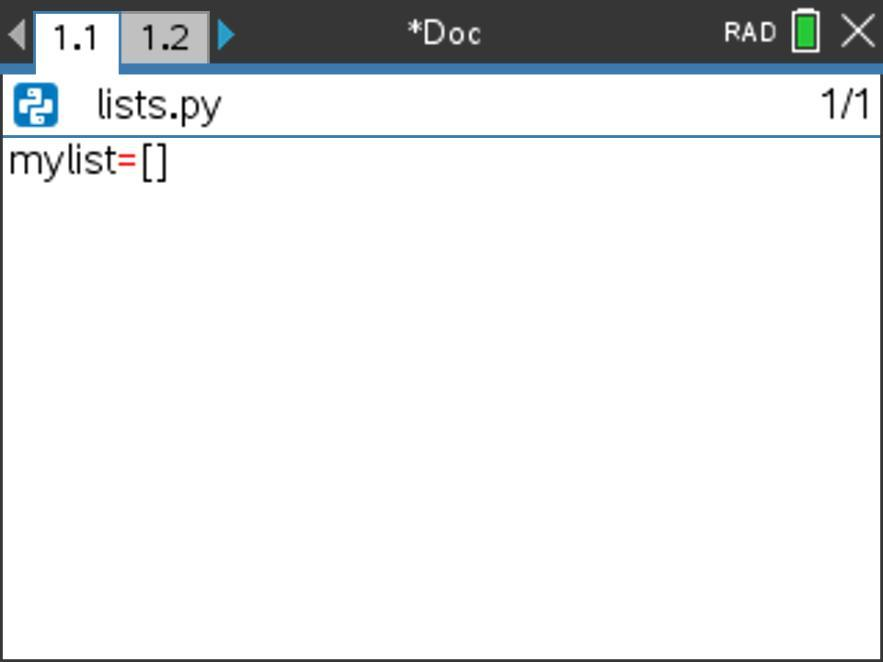Now, say we want to ask the user for data elements to go in the first five elements in this array, and then we want to save these values into their corresponding positions in the array. How might we do that? What might that code look like?

When we’re writing a program to solve a problem, it’s often helpful to write a program that answers an easier version of the problem first.

So, here, we might consider: How would we add to our program so that we ask the user for just the first element in the list and save that value in that position? If we can do that, then maybe we can expand that code to follow a similar procedure for acquiring the additional data points.

In the Python coding language on the TI-Nspire™ CX II graphing calculator, we can use an input statement to get info from the user. Let’s say we ask the user for a value for element 0. We might use syntax like this: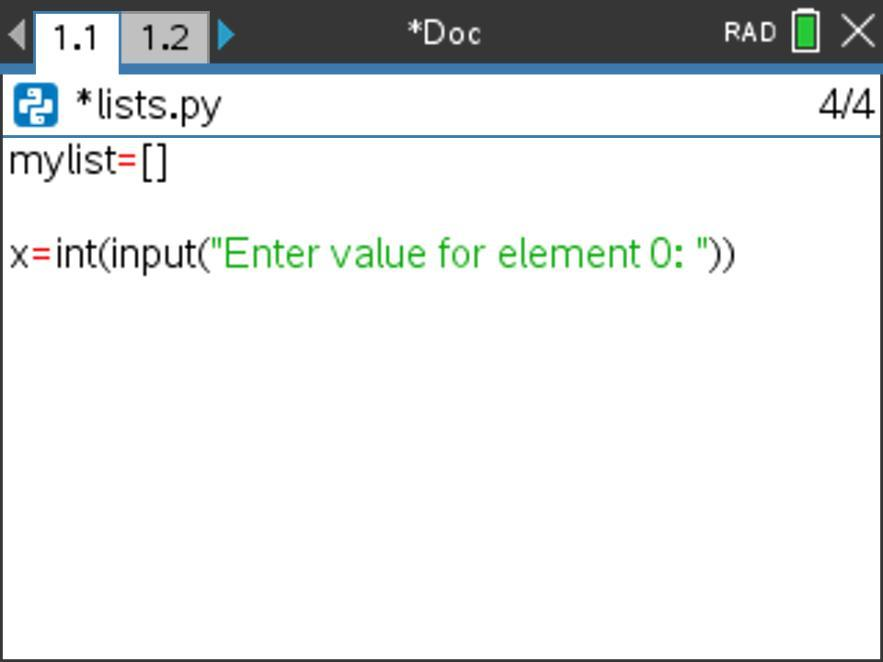The syntax for an input statement includes a message to the user (this is written in quotation marks inside a set of parentheses) and a variable location to save the user’s response to (this is before the equals sign at the beginning of the statement). Here we’ve also converted the user’s input to an integer using the int() data type command.

We’ve saved the user’s response to variable x, but what do we want to do with it? We want to add it to the end of mylist. As we saw in the last blog post, we can do that in at least three different ways. Here, let’s use the += iteration operator. (Can you think of the other two ways we could do this?)

We might then want to display mylist so that the user can see if the values have been saved to the correct positions. Let’s enter a print statement that will show this information to the user.

Let’s run our program to see if it works as desired.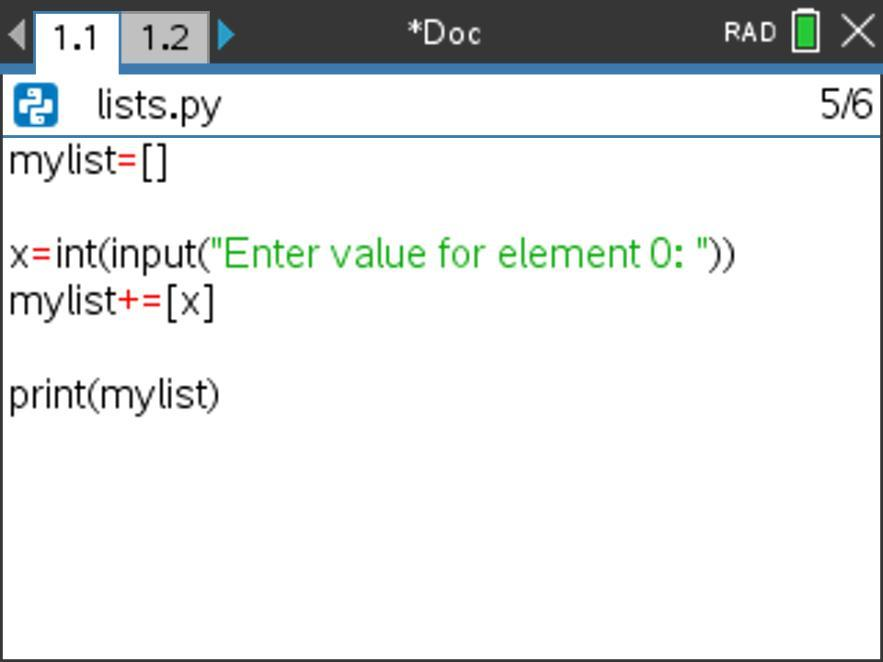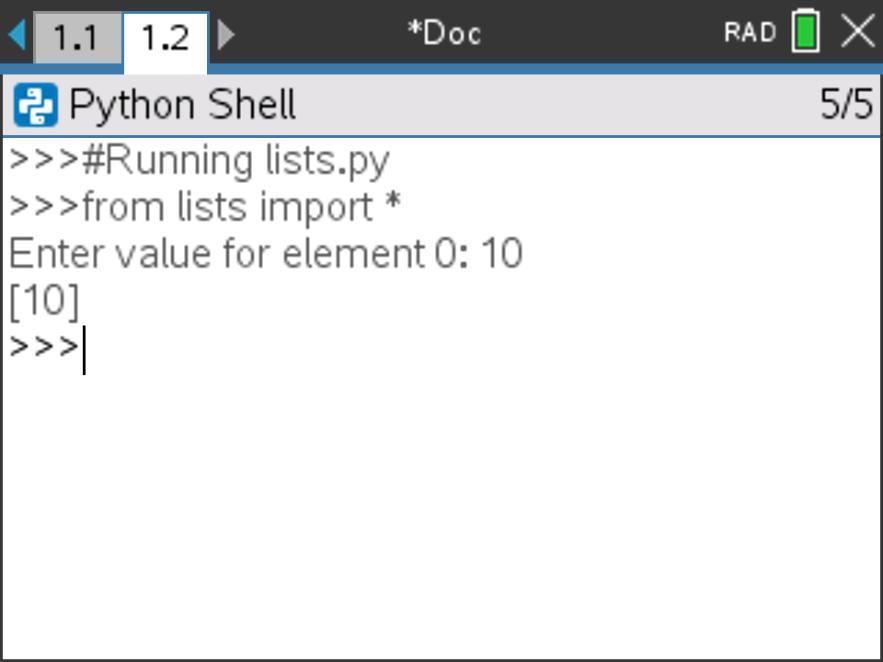Wonderful! Now, let’s extend this program to gather additional data points in the array.

We could copy and paste the previous input statement and change the values from element 0 to element 1. However, when we do that, we see that we would be using the same command again and again.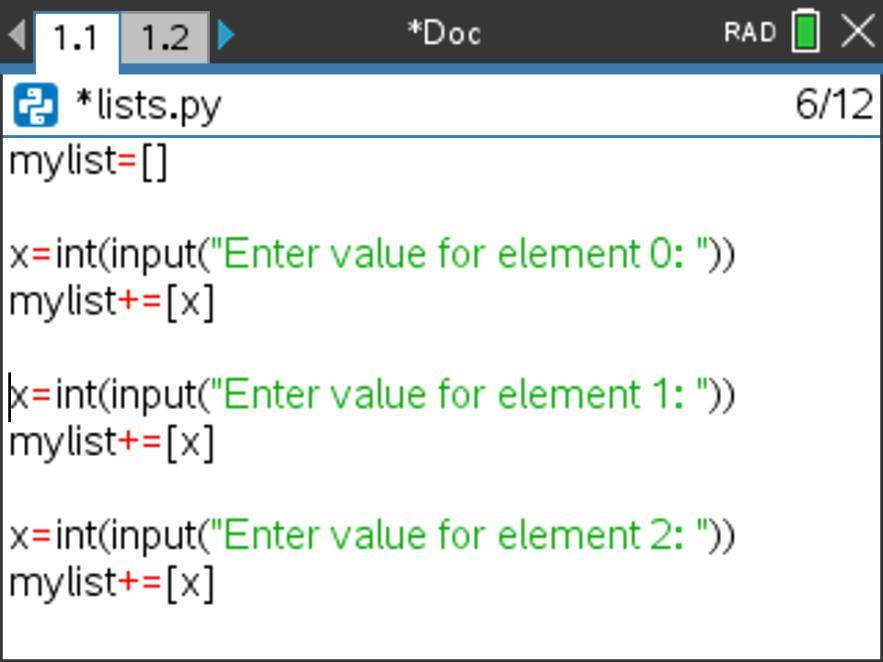As we saw earlier, when we’re doing the same thing again and again, this is a great opportunity to use a loop to simplify our code.

Let’s embed a For loop around our input statement to see if we can iterate through this command for the five array elements we want to gather and store.

The syntax for a For loop involves a loop variable (we’ll use i here), as well as an upper bound (end) value for the range command. We’ll use 5 here since we want to run this loop five times.

Consider: What else (if anything) do we need to update in the code shown below to make this program run as desired? Explain your thinking aloud to yourself or a colleague.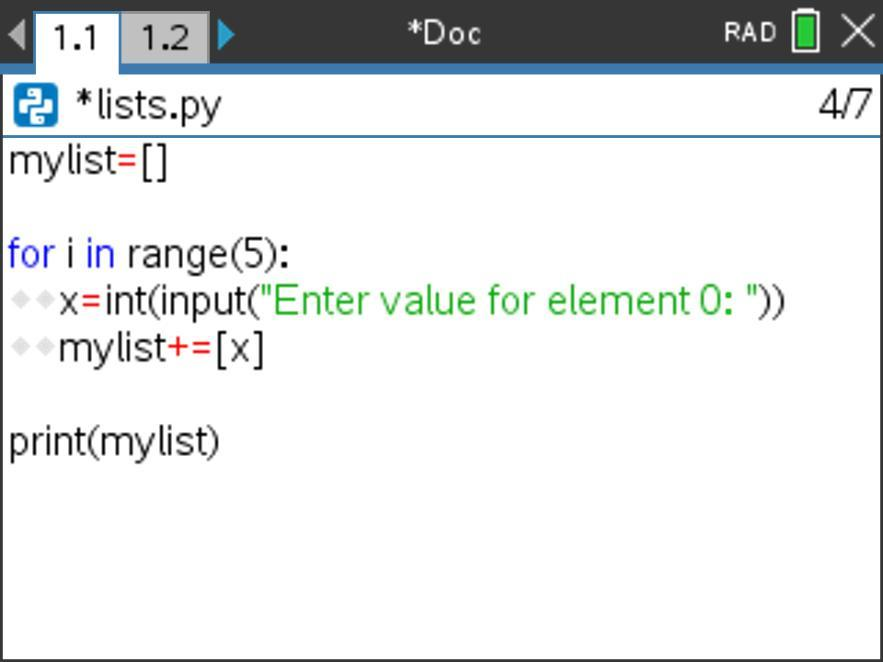We need to abstract the element number in the input statement shown to the user so that the user is asked for a different element each time.

Let’s make those changes and then run our program. Here, we’ll use concatenation (+) and string conversion (str()) to abstract the element number in the input statement that is shown to the user.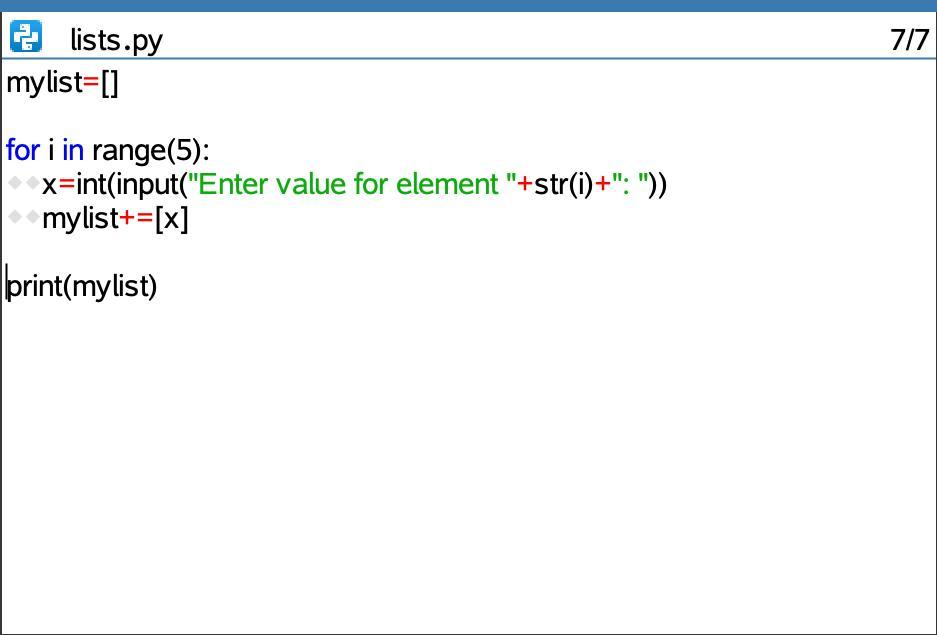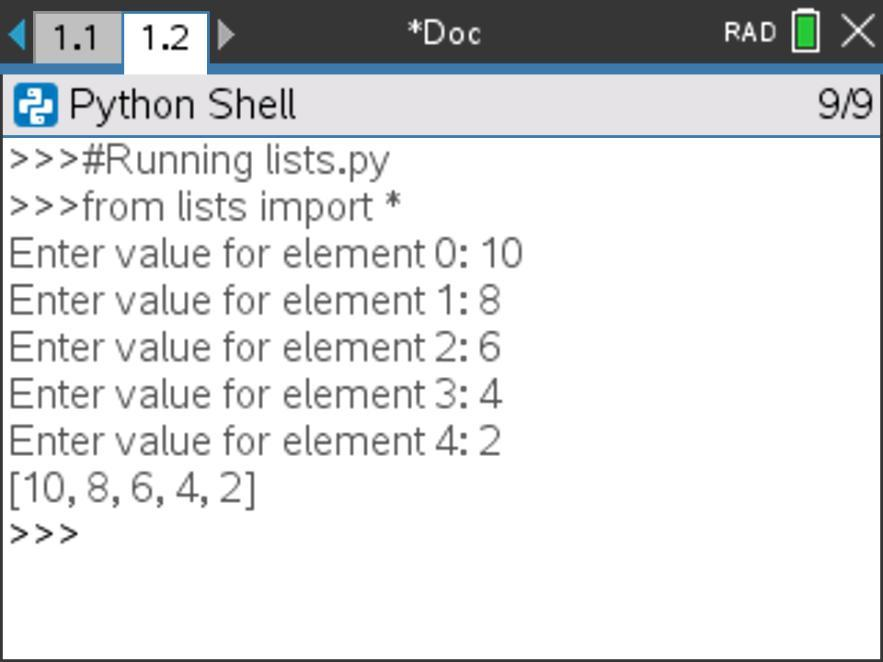## Unit 5, Activity 3: Operating on Lists

A great introduction to list operations can be found in Unit 5, Activity 3. Start by working through that activity, then read through the following discussion of main ideas from the activity.

### Entering values into a list using input

How is the x=int(input(“Enter value for element ”+str(i+1)+“:”)) line of code similar to what we did above? How is it different? What will those differences look like when the program is run?

Also, how does this line of code work? Take a minute and explain it aloud to yourself or a colleague, then read on for a sample explanation.

The input statement prompts the user for a value — specifically, the value for element i+1. i+1 is used since i starts at 0 because of the values in range(5) (which are 0, 1, 2, 3, 4).

The +str(i+1)+ concatenation updates the prompt to the user with the element number currently being prompted for.

x=int( at the beginning of the input statement stores the value inputted by the user into the variable x as an integer.

The next line of code then appends this value stored in x onto the end of the list mylist. Since the loop is incrementing by 1 from 0 to 4, the next value is always being placed in the position one place past the current end of the list.

Notice that previously we used code of mylist+=[x] to add an element to the end of a list. Here, we used mylist.append(x). Which method do you prefer? Why?

Tech tip: You can explore additional list commands from the Menu > Built-Ins > Lists submenu. These are not critical for the work in this workshop, but might be of interest and certainly connect to larger CS topics, such as sorting algorithms. You can explore these commands by creating a list in a Python shell and then operating on the initial list with these commands or by using them in a program.

The Your Turn challenge on page 2 of Unit 5, Activity 3 is an interesting exercise. We will return to it and other list challenges later on.

## Formalizing list operations

Let’s use an interactive notes page to formalize what we’ve been exploring about list operations. Before we do that, take a minute to decipher the program shown in the screenshot below.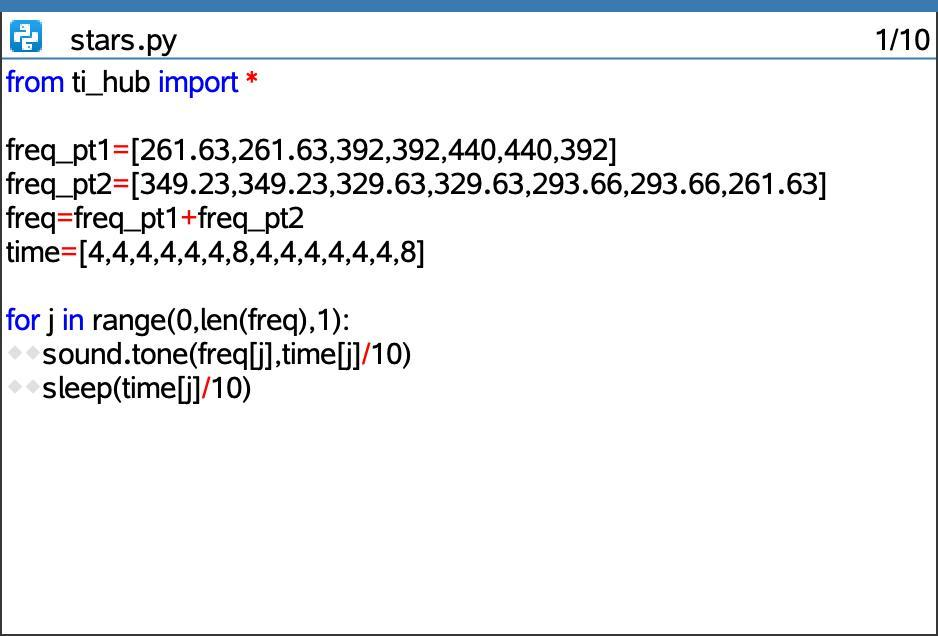This program uses the functionality of the TI-Innovator™ Hub with TI LaunchPad™ Board to produce sounds.

What do you think the output of the stars program will be when it is executed? Take a minute to trace through the loop and write down your prediction.

Here’s a short video clip of the TI-Innovator™ Hub running the program stars.

So, what was happening in this program?

At the top of the program we see input entry of frequency and time values, including augmenting two partial frequency lists (freq_pt1 and freq_pt2) into one combined frequency list (freq) using the + operator. The time values are stored in another array called time.

The For loop cycles through from element 0 through the end of the freq list. How does the program know how long the freq list is? It uses the command len(freq) to find the length of this array.

The sound.tone() command plays the frequency in element j of freq for 1/10th of the time stored in element j of array time.

Note: The desired times for the notes to play are not 4 seconds and 8 seconds as appear in array time. Rather, the desired times are 0.4 seconds and 0.8 seconds. Rather than type these values in as such, this program coded the values using integers, then divided the times within the sound.tone() and sleep() functions by 10 to convert them to their proper place value.

The sleep() command instructs the program to pause for the amount of time the note is playing before moving on to the next command.

Here are two pictures of the Working with 1D Arrays notes page designed to capture our important learnings about list operations. This notes page uses the stars program that we just considered above.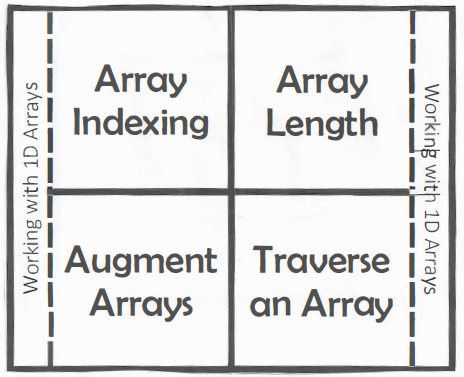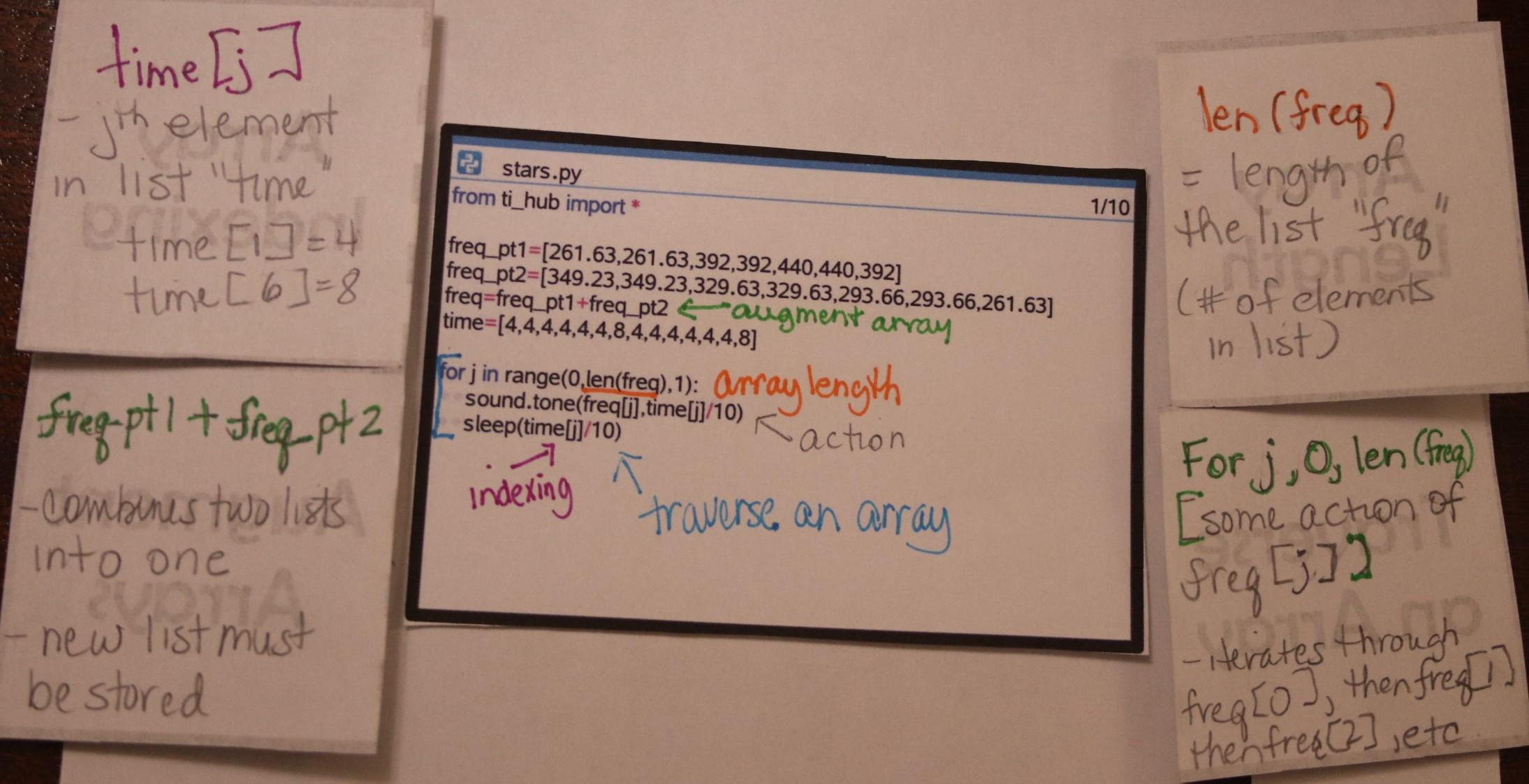## Extensions: Operating on lists

Here are five challenges to test your understanding of loops, arrays and operating on arrays.

### Array Challenge #1

Given the array list1:={10, 8, 6, 4, 2}, write a program that will output/display the values in the array from the back to the front.

### Array Challenge #2

This is the Your Turn challenge from Unit 5, Activity 3: Operating on Lists.

Write a program that would reverse the elements in an array. For example, given mylist that contains {1, 4, 3, 2}, the program would change mylist so that it contains {2, 3, 4, 1}.

Does your program work for any size array?

### Array Challenge #3

Write a program that creates a list of unknown (to the user) length and populates it randomly with values from 0–9.

Add to your program so that the program requests a value from the user, then add this value to the beginning of the list of unknown length.

### Array Challenge #4

Write a program that:

• Creates an array of length 10 where each entry in the array is a randomly determined value of 0 or 1
• Determines the number of times the value 1 appears in the array

How does this program model the number of times a person flips tails in a trial of 10 coin flips?

### Array Challenge #5

This challenge extends our thinking from one-dimensional (1D) arrays into multi-dimensional arrays.

Write a program that flattens a multi-dimensional array. In other words, the program should take a multi-dimensional array and create a one-dimensional array out of it, where the elements are in the same order they were in the original multi-dimensional array.

Here are a few hints about how to work with multi-dimensional arrays in Python on the TI-Nspire™ CX II graphing calculator: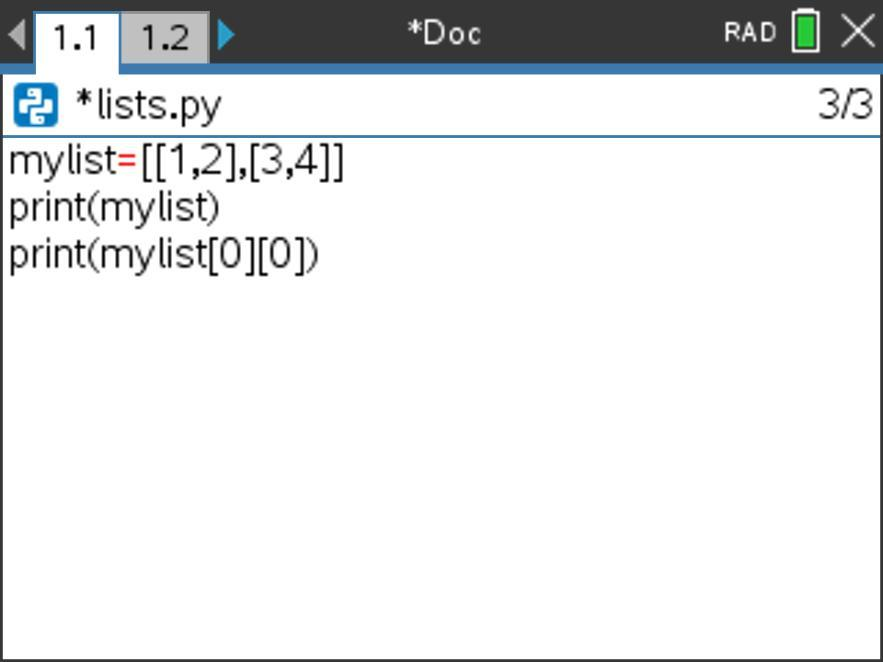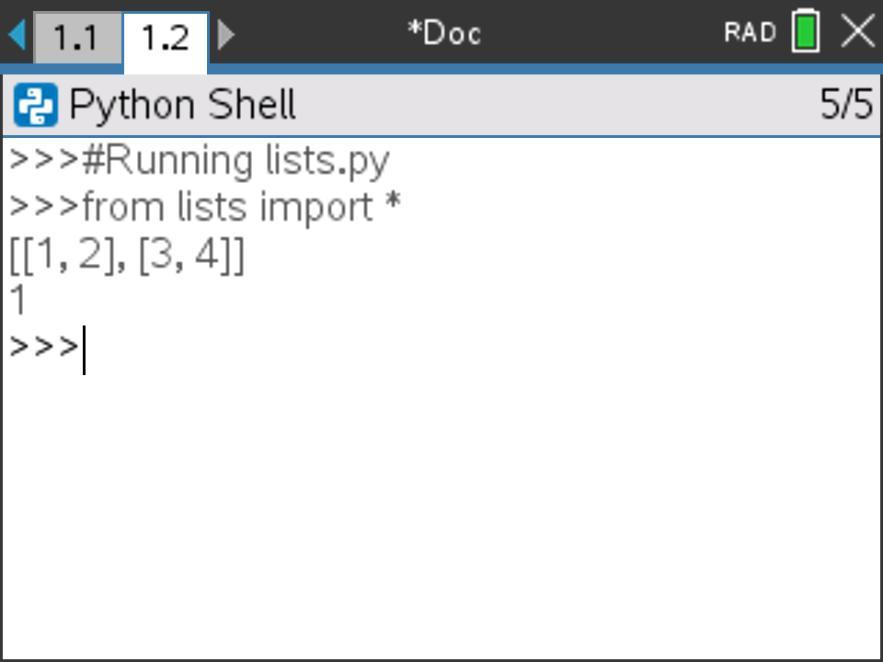## Reflecting on list operations

In this activity, we operated on lists (also known as arrays) and their elements, then used these operations to analyze lists and their elements.

Consider:

• Arrays and loops are powerful tools that are often used together. Why? How so?

This concludes this five-part activity series that extends the “Teaching Foundations of Computer Science With Python on TI-Nspire™ Technology” workshop to study additional topics from computer science with the support of the TI-Nspire™ CX II graphing calculator with Python coding technology.

This series includes: